# How to Calculate and Solve for P(A or B) | Probability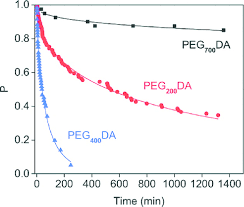The image above represents P(A or B).

To compute for P(A or B), four essential parameters are needed and these parameters are xA, NA, xB and NB.

The formula for calculating P(A or B):

P(A or B) = P(A) + P(B)

Where;

P(A) =  xANA
P(B) = xBNB

Let’s solve an example;
Find the P(A or B) when xA is 9, NA is 11, xB is 6 and NB is 12.

This implies that;

xA = 9
NA = 11
xB = 6
NB = 12

P(A or B) = P(A) + P(B)
P(A or B) = xANA + xBNB
P(A or B) = 911 + 612
P(A or B) = 9(12) + 6(11)(11)(12)
P(A or B) = 108 + 66132
P(A or B) = 174132
Dividing the numerator and denominator by 6
P(A or B) = 2922
P(A or B) = 1.318

Therefore, the P(A or B) is 1.318.

Nickzom Calculator – The Calculator Encyclopedia is capable of calculating the P(A or B).

To get the answer and workings of the P(A or B) using the Nickzom Calculator – The Calculator Encyclopedia. First, you need to obtain the app.

You can get this app via any of these means:

To get access to the professional version via web, you need to register and subscribe for NGN 1,500 per annum to have utter access to all functionalities.
You can also try the demo version via https://www.nickzom.org/calculator

Apple (Paid) – https://itunes.apple.com/us/app/nickzom-calculator/id1331162702?mt=8
Once, you have obtained the calculator encyclopedia app, proceed to the Calculator Map, then click on Probability under Mathematics.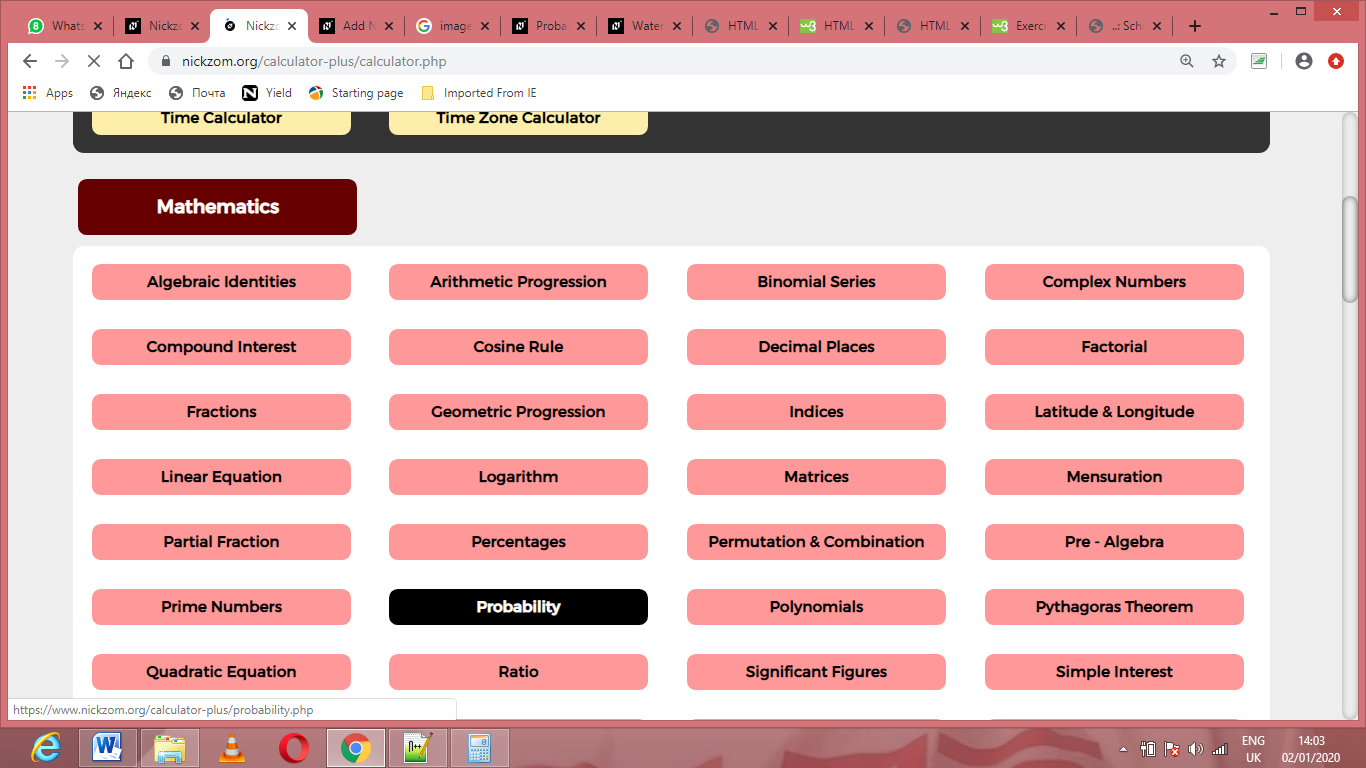Now, Click on P(A or B) under Probability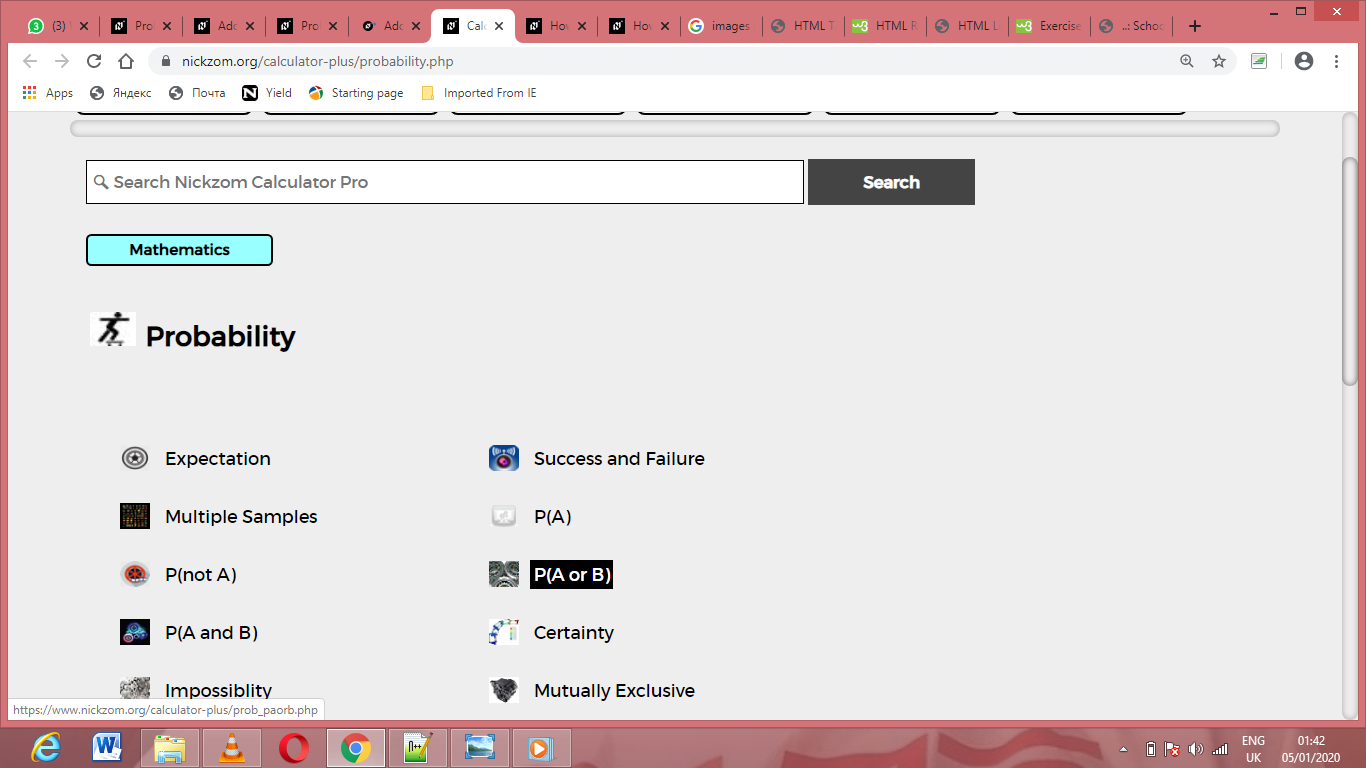The screenshot below displays the page or activity to enter your values, to get the answer for the P(A or B) according to the respective parameters which are the xA, NA, xB and NB.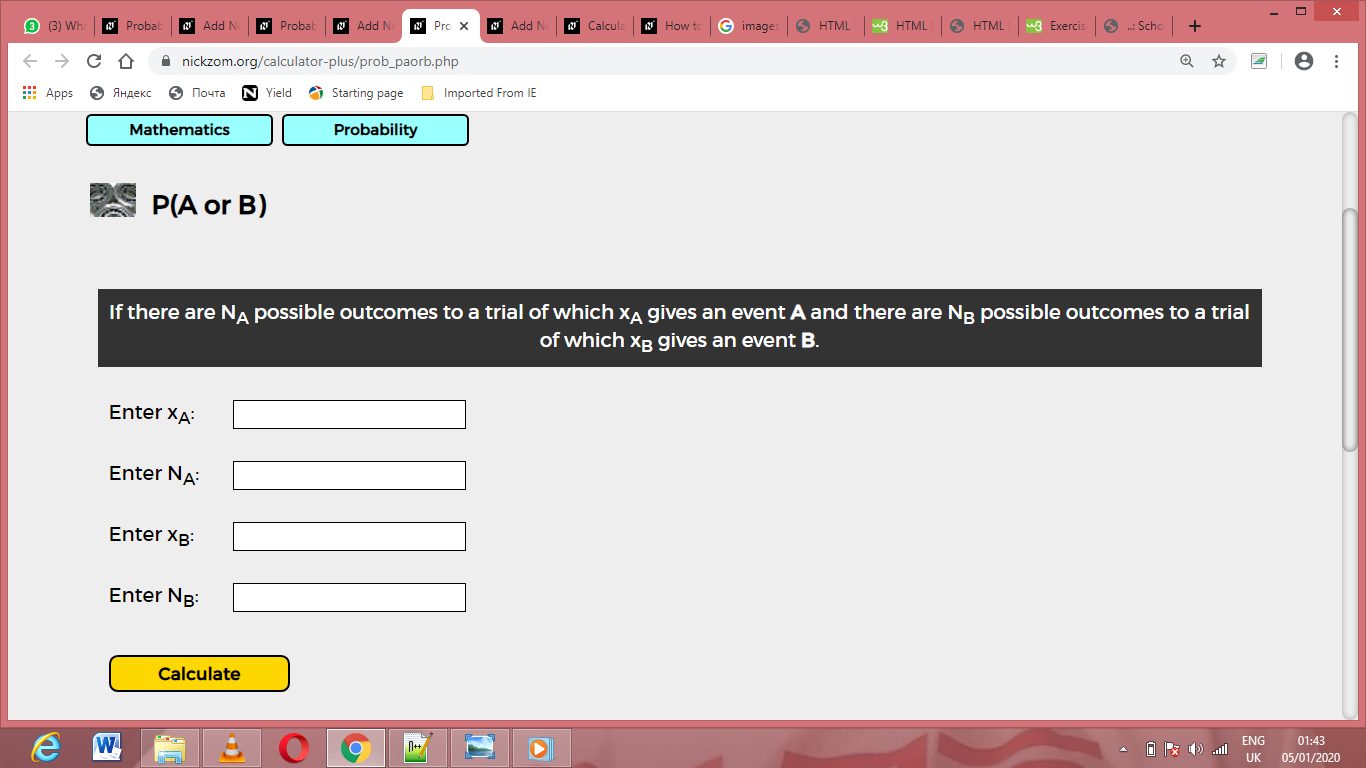Now, enter the values appropriately and accordingly for the parameters as required by the xA is 9, NA is 11, xB is 6 and NB is 12.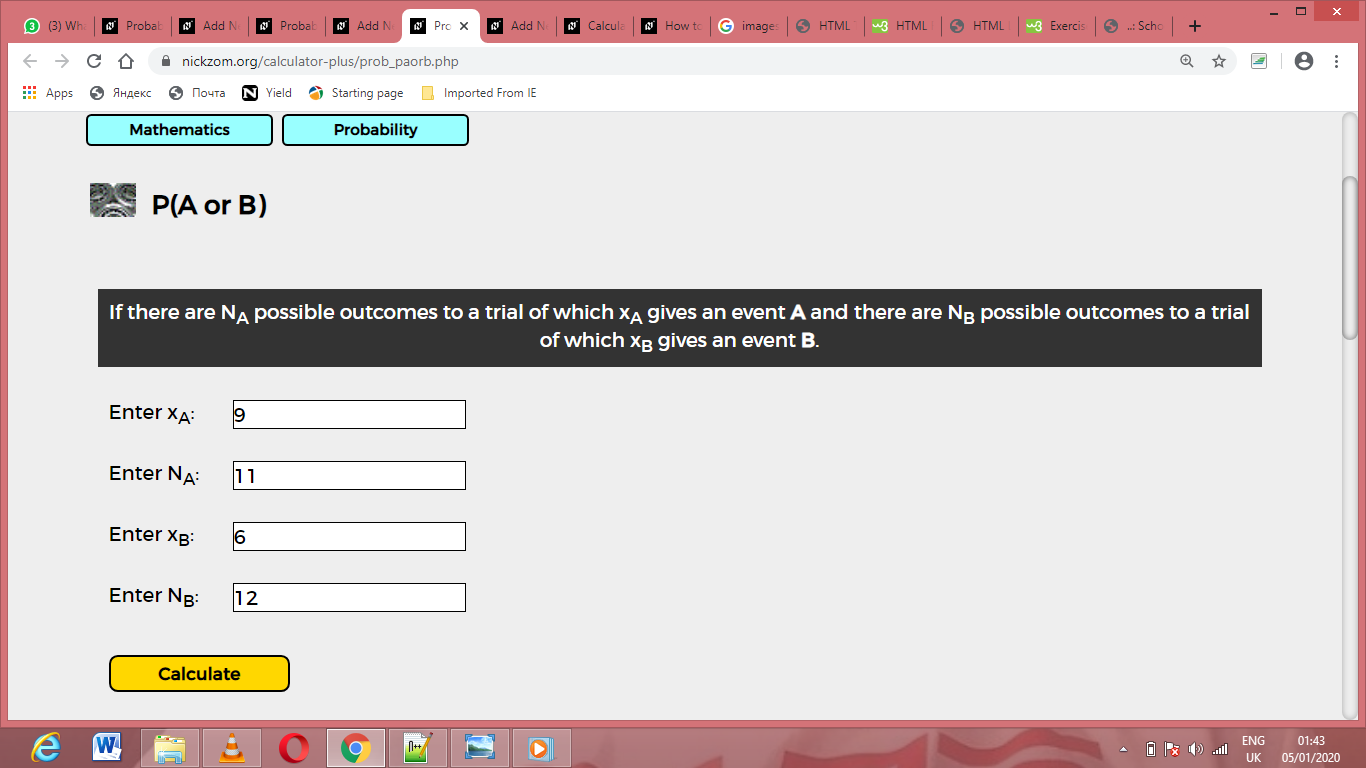Finally, Click on Calculate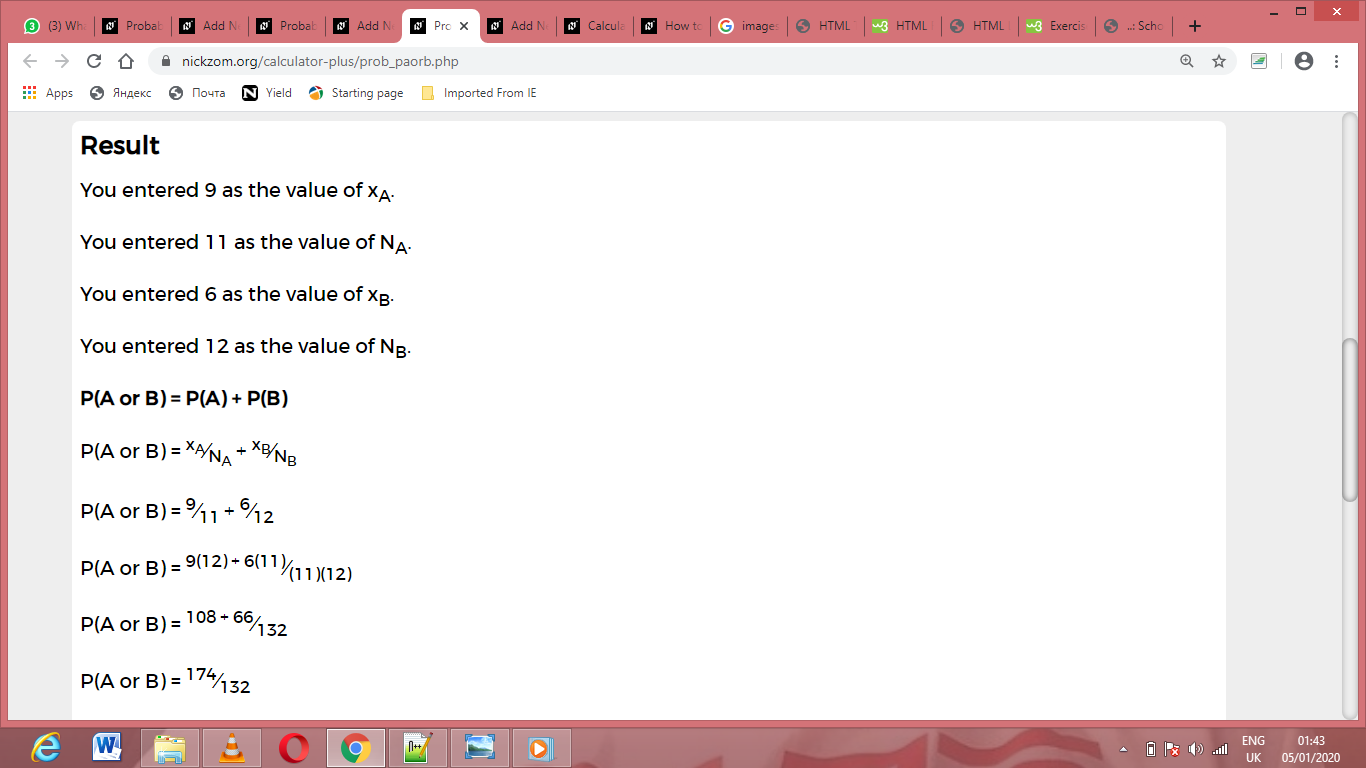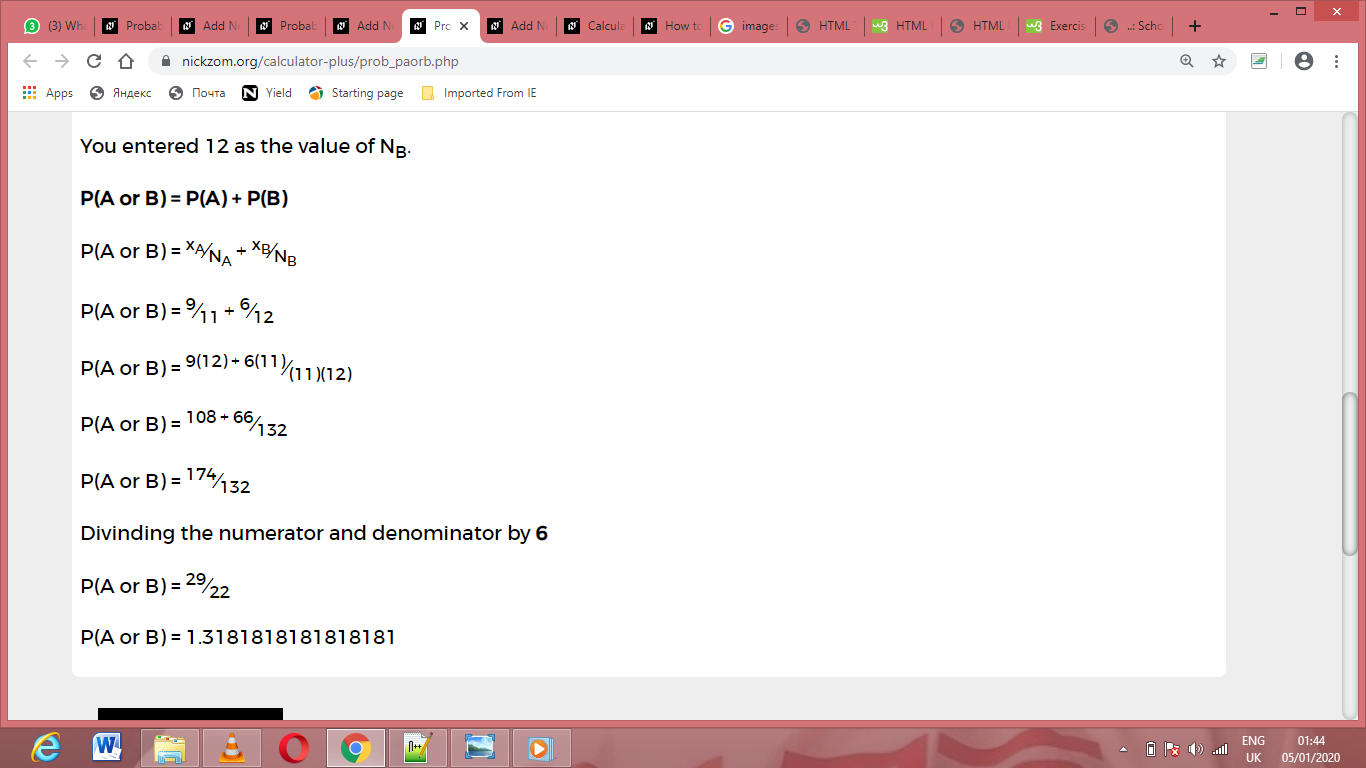As you can see from the screenshot above, Nickzom Calculator– The Calculator Encyclopedia solves for the P(A or B) and presents the formula, workings and steps too.